# Leveraging high level and low-level features for multimedia event detection.2nd.revised

12 de Apr de 2014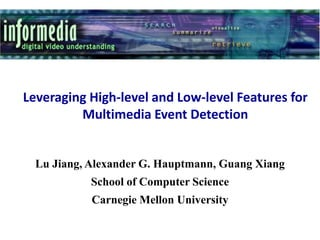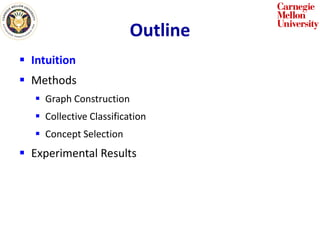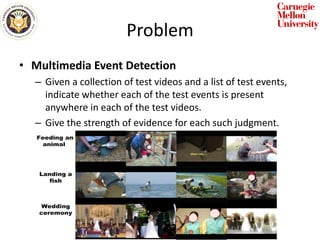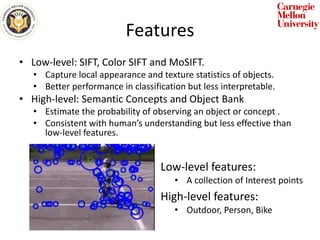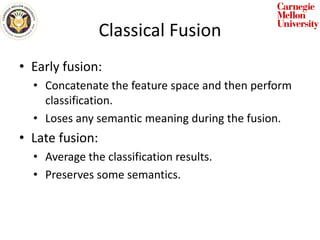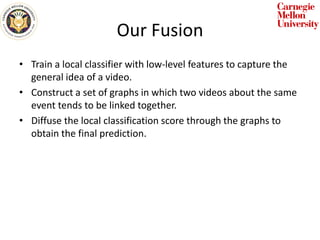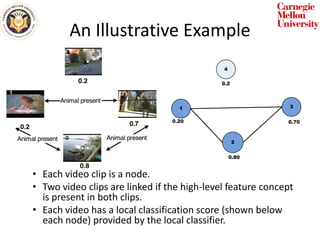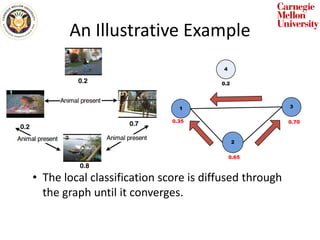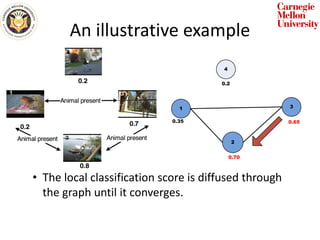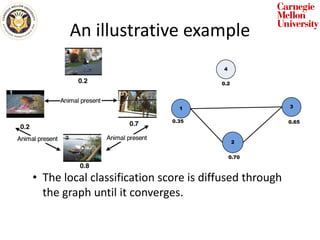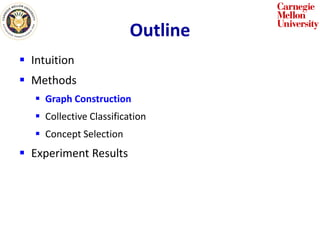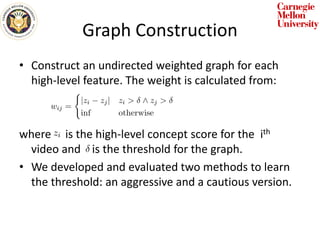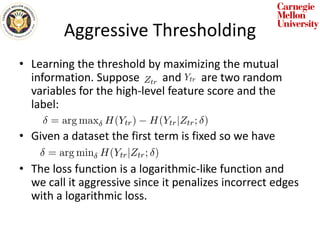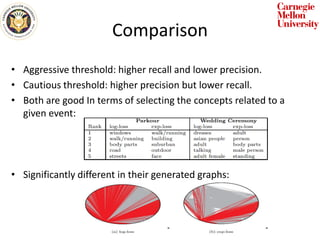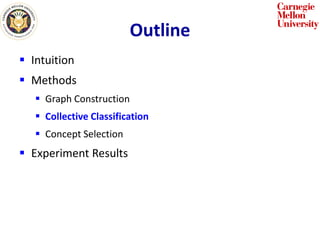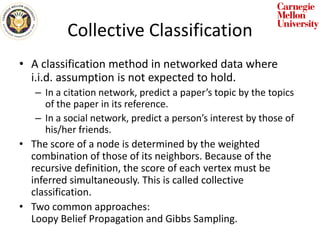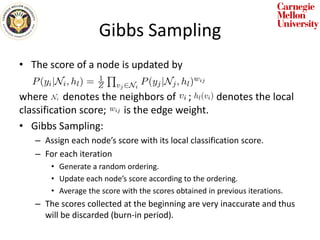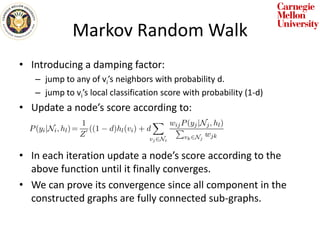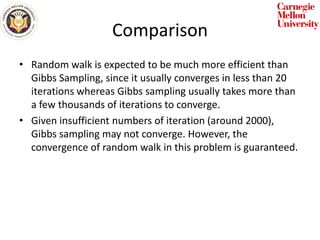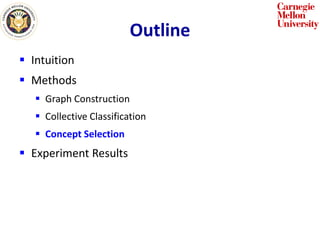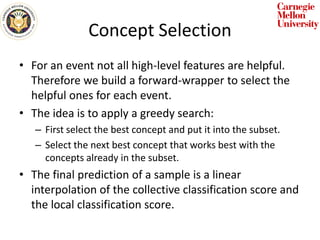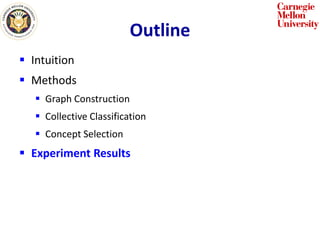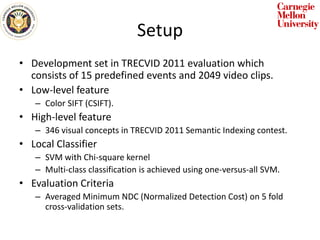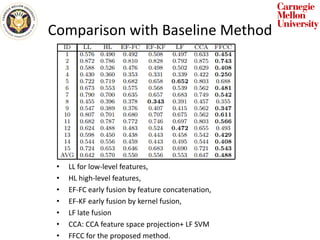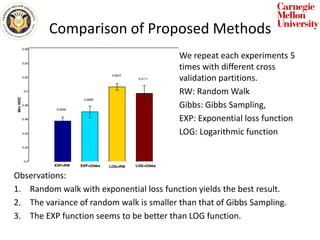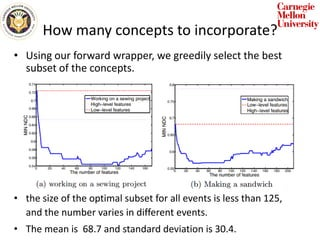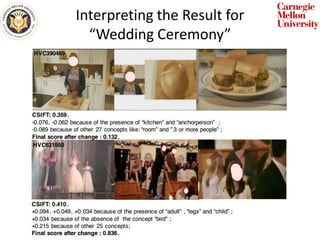1 de 29

### Leveraging high level and low-level features for multimedia event detection.2nd.revised

• 1. Leveraging High-level and Low-level Features for Multimedia Event Detection Lu Jiang, Alexander G. Hauptmann, Guang Xiang School of Computer Science Carnegie Mellon University
• 2. Outline  Intuition  Methods  Graph Construction  Collective Classification  Concept Selection  Experimental Results
• 3. Problem • Multimedia Event Detection – Given a collection of test videos and a list of test events, indicate whether each of the test events is present anywhere in each of the test videos. – Give the strength of evidence for each such judgment.
• 4. Features • Low-level: SIFT, Color SIFT and MoSIFT. • Capture local appearance and texture statistics of objects. • Better performance in classification but less interpretable. • High-level: Semantic Concepts and Object Bank • Estimate the probability of observing an object or concept . • Consistent with human’s understanding but less effective than low-level features. Low-level features: • A collection of Interest points High-level features: • Outdoor, Person, Bike
• 5. Classical Fusion • Early fusion: • Concatenate the feature space and then perform classification. • Loses any semantic meaning during the fusion. • Late fusion: • Average the classification results. • Preserves some semantics.
• 6. Our Fusion • Train a local classifier with low-level features to capture the general idea of a video. • Construct a set of graphs in which two videos about the same event tends to be linked together. • Diffuse the local classification score through the graphs to obtain the final prediction.
• 7. An Illustrative Example • Each video clip is a node. • Two video clips are linked if the high-level feature concept is present in both clips. • Each video has a local classification score (shown below each node) provided by the local classifier. 2 3 4 1 0.2 0.80 0.20 0.70
• 8. An Illustrative Example • The local classification score is diffused through the graph until it converges. 2 3 4 1 0.2 0.65 0.35 0.70
• 9. An illustrative example • The local classification score is diffused through the graph until it converges. 2 3 4 1 0.2 0.70 0.35 0.65
• 10. An illustrative example • The local classification score is diffused through the graph until it converges. 2 3 4 1 0.2 0.70 0.35 0.65
• 11. Outline  Intuition  Methods  Graph Construction  Collective Classification  Concept Selection  Experiment Results
• 12. Graph Construction • Construct an undirected weighted graph for each high-level feature. The weight is calculated from: where is the high-level concept score for the ith video and is the threshold for the graph. • We developed and evaluated two methods to learn the threshold: an aggressive and a cautious version.
• 13. Aggressive Thresholding • Learning the threshold by maximizing the mutual information. Suppose and are two random variables for the high-level feature score and the label: • Given a dataset the first term is fixed so we have • The loss function is a logarithmic-like function and we call it aggressive since it penalizes incorrect edges with a logarithmic loss.
• 14. Cautious Thresholding • Learning the threshold minimizing the following loss function: • where counts the number of incorrectly connected samples and imposes a significant penalty when becomes larger. • The loss function is an exponential like function.
• 15. Comparison • Aggressive threshold: higher recall and lower precision. • Cautious threshold: higher precision but lower recall. • Both are good In terms of selecting the concepts related to a given event: • Significantly different in their generated graphs:
• 16. Outline  Intuition  Methods  Graph Construction  Collective Classification  Concept Selection  Experiment Results
• 17. Collective Classification • A classification method in networked data where i.i.d. assumption is not expected to hold. – In a citation network, predict a paper’s topic by the topics of the paper in its reference. – In a social network, predict a person’s interest by those of his/her friends. • The score of a node is determined by the weighted combination of those of its neighbors. Because of the recursive definition, the score of each vertex must be inferred simultaneously. This is called collective classification. • Two common approaches: Loopy Belief Propagation and Gibbs Sampling.
• 18. Gibbs Sampling • The score of a node is updated by where denotes the neighbors of ; denotes the local classification score; is the edge weight. • Gibbs Sampling: – Assign each node’s score with its local classification score. – For each iteration • Generate a random ordering. • Update each node’s score according to the ordering. • Average the score with the scores obtained in previous iterations. – The scores collected at the beginning are very inaccurate and thus will be discarded (burn-in period).
• 19. Markov Random Walk • Introducing a damping factor: – jump to any of vi’s neighbors with probability d. – jump to vi’s local classification score with probability (1-d) • Update a node’s score according to: • In each iteration update a node’s score according to the above function until it finally converges. • We can prove its convergence since all component in the constructed graphs are fully connected sub-graphs.
• 20. Comparison • Random walk is expected to be much more efficient than Gibbs Sampling, since it usually converges in less than 20 iterations whereas Gibbs sampling usually takes more than a few thousands of iterations to converge. • Given insufficient numbers of iteration (around 2000), Gibbs sampling may not converge. However, the convergence of random walk in this problem is guaranteed.
• 21. Outline  Intuition  Methods  Graph Construction  Collective Classification  Concept Selection  Experiment Results
• 22. Concept Selection • For an event not all high-level features are helpful. Therefore we build a forward-wrapper to select the helpful ones for each event. • The idea is to apply a greedy search: – First select the best concept and put it into the subset. – Select the next best concept that works best with the concepts already in the subset. • The final prediction of a sample is a linear interpolation of the collective classification score and the local classification score.
• 23. Outline  Intuition  Methods  Graph Construction  Collective Classification  Concept Selection  Experiment Results
• 24. Setup • Development set in TRECVID 2011 evaluation which consists of 15 predefined events and 2049 video clips. • Low-level feature – Color SIFT (CSIFT). • High-level feature – 346 visual concepts in TRECVID 2011 Semantic Indexing contest. • Local Classifier – SVM with Chi-square kernel – Multi-class classification is achieved using one-versus-all SVM. • Evaluation Criteria – Averaged Minimum NDC (Normalized Detection Cost) on 5 fold cross-validation sets.
• 25. Comparison with Baseline Method • LL for low-level features, • HL high-level features, • EF-FC early fusion by feature concatenation, • EF-KF early fusion by kernel fusion, • LF late fusion • CCA: CCA feature space projection+ LF SVM • FFCC for the proposed method.
• 26. Comparison of Proposed Methods We repeat each experiments 5 times with different cross validation partitions. RW: Random Walk Gibbs: Gibbs Sampling, EXP: Exponential loss function LOG: Logarithmic function Observations: 1. Random walk with exponential loss function yields the best result. 2. The variance of random walk is smaller than that of Gibbs Sampling. 3. The EXP function seems to be better than LOG function.
• 27. How many concepts to incorporate? • Using our forward wrapper, we greedily select the best subset of the concepts. • the size of the optimal subset for all events is less than 125, and the number varies in different events. • The mean is 68.7 and standard deviation is 30.4.
• 28. Interpreting the Result for “Wedding Ceremony”